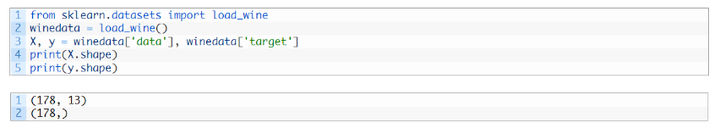# PCA主成分分析Python实现

+关注继续查看

Github源码：https://github.com/csuldw/MachineLearning/tree/master/PCA

PCA（principle component analysis） ，主成分分析，主要是用来降低数据集的维度，然后挑选出主要的特征。原理简单，实现也简单。关于原理公式的推导，本文不会涉及，你可以参考下面的参考文献，也可以去Wikipedia，这里主要关注实现，算是锻炼一下自己，对PCA在理论的基础上画个圆满的句号。

PCA思想

LDA思想：最大类间距离，最小类内距离。简而言之，第一，为了实现投影后的两个类别的距离较远，用映射后两个类别的均值差的绝对值来度量。第二，为了实现投影后，每个类内部数据点比较聚集，用投影后每个类别的方差来度量。

LDA is also closely related to principal component analysis (PCA) and factor analysis in that they both look for linear combinations of variables which best explain the data. LDA explicitly attempts to model the difference between the classes of data. PCA on the other hand does not take into account any difference in class, and factor analysis builds the feature combinations based on differences rather than similarities. Discriminant analysis is also different from factor analysis in that it is not an interdependence technique: a distinction between independent variables and dependent variables (also called criterion variables) must be made.

1.首先引入numpy，由于测试中用到了pandas和matplotlib，所以这里一并加载

 1 2 3 import numpy as np import pandas as pd import matplotlib.pyplot as plt

2.定义一个均值函数

#计算均值,要求输入数据为numpy的矩阵格式，行表示样本数，列表示特征

 1 2 def meanX(dataX):     return np.mean(dataX,axis=0)#axis=0表示按照列来求均值，如果输入list,则axis=1

3.编写pca方法，具体解释参考注释

 1 2 3 4 5 6 7 8 9 10 11 12 13 14 15 16 17 18 19 20 21 22 23 24 25 26 27 """ 参数： - XMat：传入的是一个numpy的矩阵格式，行表示样本数，列表示特征     - k：表示取前k个特征值对应的特征向量 返回值： - finalData：参数一指的是返回的低维矩阵，对应于输入参数二 - reconData：参数二对应的是移动坐标轴后的矩阵 """ def pca(XMat, k):     average = meanX(XMat)      m, n = np.shape(XMat)     data_adjust = []     avgs = np.tile(average, (m, 1))     data_adjust = XMat - avgs     covX = np.cov(data_adjust.T)   #计算协方差矩阵     featValue, featVec=  np.linalg.eig(covX)  #求解协方差矩阵的特征值和特征向量     index = np.argsort(-featValue) #按照featValue进行从大到小排序     finalData = []     if k > n:         print "k must lower than feature number"         return     else:         #注意特征向量时列向量，而numpy的二维矩阵(数组)a[m][n]中，a表示第1行值         selectVec = np.matrix(featVec.T[index[:k]]) #所以这里需要进行转置         finalData = data_adjust * selectVec.T          reconData = (finalData * selectVec) + average       return finalData, reconData

4.编写一个加载数据集的函数

#输入文件的每行数据都以\t隔开

 1 2 def loaddata(datafile):     return np.array(pd.read_csv(datafile,sep="\t",header=-1)).astype(np.float)

5.可视化结果

 1 2 3 4 5 6 7 8 9 10 11 12 13 14 15 16 17 18 19 20 21 def plotBestFit(data1, data2):       dataArr1 = np.array(data1)     dataArr2 = np.array(data2)           m = np.shape(dataArr1)     axis_x1 = []     axis_y1 = []     axis_x2 = []     axis_y2 = []     for i in range(m):         axis_x1.append(dataArr1[i,0])         axis_y1.append(dataArr1[i,1])         axis_x2.append(dataArr2[i,0])          axis_y2.append(dataArr2[i,1])       fig = plt.figure()     ax = fig.add_subplot(111)     ax.scatter(axis_x1, axis_y1, s=50, c='red', marker='s')     ax.scatter(axis_x2, axis_y2, s=50, c='blue')     plt.xlabel('x1'); plt.ylabel('x2');     plt.savefig("outfile.png")     plt.show()

6.测试方法

data.txt可到github中下载：data.txt

#根据数据集data.txt

 1 2 3 4 5 6 7 8 def main():         datafile = "data.txt"     XMat = loaddata(datafile)     k = 2     return pca(XMat, k) if __name__ == "__main__":     finalData, reconMat = main()     plotBestFit(finalData, reconMat)Wikipedia- Linear discriminant analysis

 Wikipedia- Principal_component_analysis

知乎-如何理解矩阵特征值

http://www.csuldw.com/2016/02/28/2016-02-28-pca/PCA主成分分析的可视化（Python）

132 0Python - 爬虫中文乱码之请求头 Accept-Encoding Brotli 问题
Python - 爬虫中文乱码之请求头 Accept-Encoding Brotli 问题
51 0【安全合规】python爬虫从0到1 -ajax的get请求进阶
python爬虫从0到1 -ajax的get请求进阶
27 0【安全合规】python爬虫从0到1 -ajax的get请求

28 0Python爬虫：scrapy-splash的请求头和代理参数设置
Python爬虫：scrapy-splash的请求头和代理参数设置
123 0Python爬虫：常用的user_agent请求头
Python爬虫：常用的user_agent请求头
84 054 0Python爬虫，用第三方库解决下载网页中文本的问题

1073 0
23704

0